Part 1: Linear Algebra Assessment
Email *
Full Name *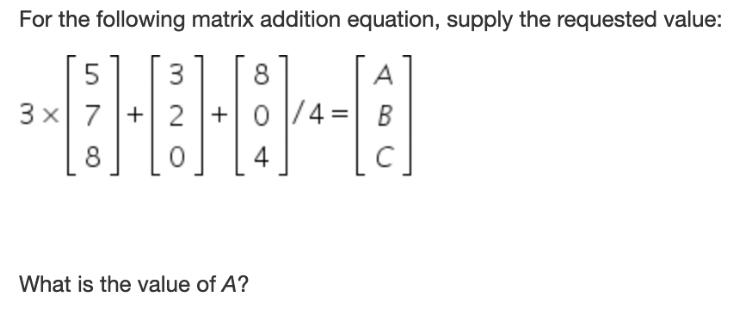Matrix-Vector Multiplication *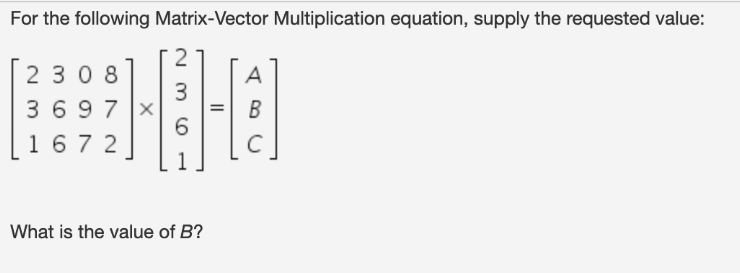Matrix-Matrix Multiplication *Properties of Matrix Multiplication 1 *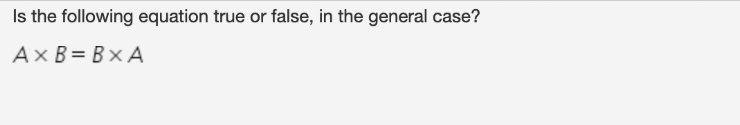Properties of Matrix Multiplication 2 *Matrix Inverse *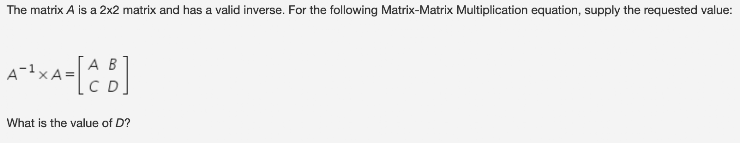Matrix Transpose *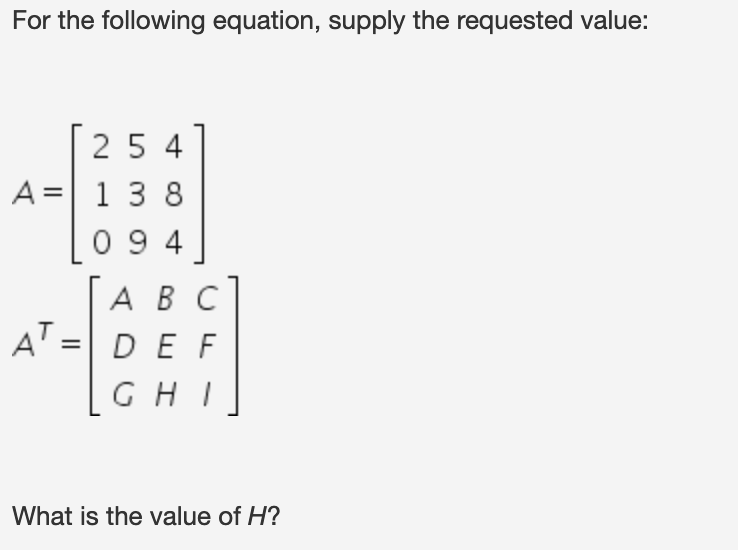Part 2: Probability & Statistics
Calculating Probabilities #1: A bag contains 2 orange, 3 purple and 2 yellow balls. Two of the balls are randomly drawn. What is the probability that none of the balls drawn is yellow? Answer to three significant digits. *
Calculating Probabilities #2: A normal deck of cards has 52 cards in it. Assuming that you DON'T replace the card you had drawn before the next draw, what is the probability that you will draw three kings in a row? Answer to three significant digits. *
Bayes Theorem #1: Julie is getting married tomorrow, in the desert at an outdoor ceremony. It usually only rains 8 days each year. Unfortunately, rain is predicted for tomorrow by the local weatherman. When it does rains, the weatherman correctly forecasts rain 92% of the time. When it doesn't rain, he incorrectly forecasts rain 14% of the time. What is the probability that on the day of Julie's wedding it will rain? Provide your answer to three significant digits. *
Bayes Theorem #2: A specific virus infects one in every 500 people. A test used to detect the virus in an individual is positive 91% of the time if the individual has the virus and 4% of the time if the individual does not have the virus. (This 4% result is called a false positive result). Let A be the event "the individual has the virus" and B be the event "the individual tests positive". Find the probability that an individual is infected with the virus given the fact that they have just tested positive. Round your answer to three significant digits. *
A copy of your responses will be emailed to the address you provided.
Submit
Clear form